SSAT Elementary Level Math : How to find the whole from the part

Example Questions

Example Question #7 : Compare Two Three Digit Numbers: Ccss.Math.Content.2.Nbt.A.4

Which statement is true?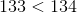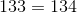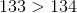There is no true statementExplanation:

The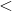sign means less than.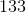is less than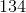because of the number in the ones place.has a lesser value than, which makesis less thanExample Question #8 : Compare Two Three Digit Numbers: Ccss.Math.Content.2.Nbt.A.4

Which statement is true?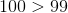There is no true statement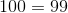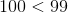Explanation:

The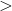sign means greater than. Of the options above,is greater than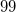Example Question #9 : Compare Two Three Digit Numbers: Ccss.Math.Content.2.Nbt.A.4

Which statement is true?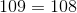There is no true statement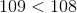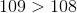Explanation:

Thesign means greater than.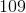is greater than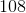because of the number in the ones place.has a greater value than, which makesgreater thanExample Question #10 : Compare Two Three Digit Numbers: Ccss.Math.Content.2.Nbt.A.4

Which statement is true?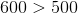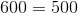There is no true statement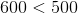Explanation:

Thesign means greater than.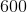is greater than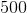because of the number in the hundreds place.has a greater value than, which makesgreater thanExample Question #1 : Count, Read, And Write Numbers 1 120: Ccss.Math.Content.1.Nbt.A.1

What number is one hundred twenty?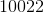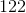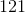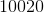Explanation:

In number form, one hundred twenty is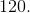Example Question #2 : Count, Read, And Write Numbers 1 120: Ccss.Math.Content.1.Nbt.A.1

What number is ninety-nine?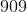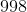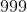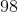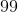Explanation:

In number form, ninety-nine is.

Example Question #3 : Count, Read, And Write Numbers 1 120: Ccss.Math.Content.1.Nbt.A.1

What number is twenty-three?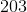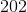Explanation:

In number form, twenty-three is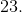Example Question #4 : Count, Read, And Write Numbers 1 120: Ccss.Math.Content.1.Nbt.A.1

What number is forty-two?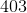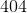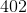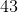Explanation:

In number form, forty-two is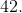Example Question #5 : Count, Read, And Write Numbers 1 120: Ccss.Math.Content.1.Nbt.A.1

What number is nineteen?Explanation:

In number form, nineteen is.

Example Question #6 : Count, Read, And Write Numbers 1 120: Ccss.Math.Content.1.Nbt.A.1

What number is one hundred thirteen?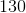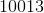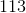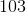In number form, one hundred thirteen is.